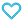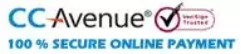# A Problem book in Mathematical Analysis

180.00

This book is useful for JEE Main/ Advanced and UG & PG students. It contains series of chapter wise topic wise questions along with answers. It will help the students studying mathematical analysis in higher educational institutions. Appendix is given at the end of the book for getting important values.

931 in stock

Qty:Add to WishlistTable of Content:
• Preface
• Chapter I. Function
• 1. Preliminaries
• 2. Simplest Properties of Functions
• 3. Basic Elementary Functions
• 4. Inverse Function. Power, Exponential and Logarithmic Functions
• 5. Trigonometric and Inverse Trigonometric Functions
• 6. Computational Problems
• Chapter II. Limit. Continuity
• 1. Basic Definitions
• 2. Infinite Magnitudes. Tests for the Existence of the Limit
• 3. Continuous Functions
• 4. Finding Limits. Comparison of Infinitesimals
• Chapter III.Derivative and Differential. Differential Calculus
• 1. Derivative. The Rate of Change of a Function
• 2. Differentiating Functions
• 3. Differential. Differentiability of a Function
• 4. The Derivative as the Rate of Change
• 5. Repeated Differentiation
• Chapter IV. Investigating Functions and Their Graphs
• 1. Behaviour of a Function
• 2. Application of the First Derivative
• 3. Application of the Second Derivative
• 4. Additional Items. Solving Equations
• 5. Taylor’s Formula and Its Application
• 6. Curvature
• 7. Computational Problems
• Chapter V. The Definite Integral
• 1. The Definite Integral and Its Simplest Properties
• 2. Basic Properties of the Definite Integral
• Chapter VI.Indefinite Integral. Integral Calculus
• 1. Simplest Integration Rules
• 2. Basic Methods of Integration
• 3. Basic Classes of Integrable Functions
• Chapter VII.Methods for Evaluating Definite Integrals.
•            Improper Integrals
• 1. Methods for Exact Evaluation of Integrals
• 2. Approximate Methods
• 3. Improper Integrals
• Chapter VIII. Application of Integral Calculus
• 1. Some Problems in Geometry and Statics
• 2. Some Physics Problems
• Chapter IX. Series
• 1. Numerical Series
• 2. Functional Series
• 3. Power Series
• 4. Some Applications of Taylor’s Series
• Chapter X. Functions of Several Variables. Differential Calculus
• 1. Functions of Several Variables
• 2. Simplest Properties of Functions
• 3. Derivatives and Differentials of Functions of Several Variables
• 4. Differentiating Functions
• 5. Repeated Differentiation
• Chapter XI. Applications of Differential Calculus of
•          Functions of Several Variables
• 1. Taylor’s Formula. Extrema of Functions of Several Variables
• 2. Plane Curves
• 3. Vector Function of a Scalar Argument. Space Curves.Surfaces.
• 4. Scalar Field.Gradient. Directional Derivative
• Chapter XII. Multiple Integrals
• 1. Double and Triple Integrals
• 2. Multiple Integration
• 3. Integrals in Polar, Cylindrical and Spherical Coordinates.
• 4. Application of Double and Triple Integrals
• 5. Improper Integrals. Integrals Dependent on Parameters
• Chapter XIII. Line Integrals and Surface Integrals
• 1. Line Integrals with Respect to Arc Length
• 2. Line Integrals with Respect to Coordinates
• 3. Surface Integrals
• Chapter XIV. Differential Equations
• 1. Equations of the First Order
• 2. General Differential Equations of the First Order
• 3. Equations of the Second and Higher Orders
• 4. Linear Equations
• 5. Systems of Differential Equations
• 6. Computational Problems
• Chapter XV. Trigonometric Series
• 1. Trigonometric Polynomials
• 2. Fourier Series
• 3. Krylov’s Method. Harmonic Analysis
• Chapter XVI. Elements of Field Theory